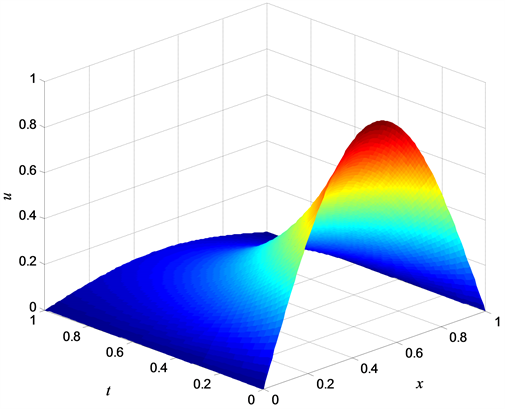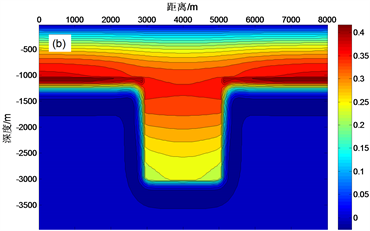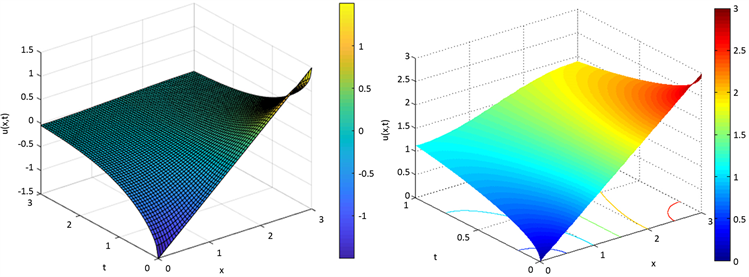# “地球物理特殊方程”课程的教学模式探索与实践Exploring and Practice of Teaching Mode on the Course of Geophysical Special Equations

DOI: 10.12677/CES.2018.66081, PDF, HTML, XML, 下载: 735  浏览: 1,810  科研立项经费支持

Abstract: The “Special Equations of Geophysics” is a basic course specialized for the discipline of geophysics in Central South University. It is also a bridge between mathematics basic courses and geophysical professional courses. The subject of this course is a partial differential equation with geophysical application background. It is characterized by organically combining mathematical theory, geophysical methods and practical applications, which is a difficult course to teach and learn. Through the design of the syllabus and teaching content and the establishment of teaching ideas, this paper conducts a series of explorations on classroom teaching and simulation practice teaching in this course, and proposes new teaching methods and means.

1. 引言

“地球物理特殊方程”包含了大量的数学描述、抽象化的数学方法、理论及公式，是大家公认的一门难教难学的课程。课程内容涉及范畴较宽、内容繁多，甚至一些章节内容本身就是一门独立的课程、自成体系，如“有限差分法”、“有限单元法”、“地球物理特殊函数”。另外，随着一些新的高精度、高效率的数值模拟算法的出现和地球物理新理论、新方法、新技术的提出，“地球物理特殊方程”又需要不断发展和充实  。本课程不仅是使学生获取一定的数学基础理论、基本知识和运算能力，而更重要的是培养学生今后的地球物理科学素质，发展学生的创新能力，培育学生的创新精神。同时，本课程对后续地球物理专业课程的学习起到非常重要的作用，且教学质量直接关系到研究生阶段的教育。围绕教学大纲、教学效果和课程建设，笔者在教学内容设计、教学思路的建立以及教学方法和教学技术的开展等方面积极实践，取得了一些实践认识。

2. 课程定位与教学内容Table 1. Teaching content and time arrangement of the special equation of geophysics (2016 Syllabus)

3. 课程教学方法与教学活动

3.1. 利用多媒体手段，增强物理过程和解的可视化

$\left\{\begin{array}{l}\frac{\partial u}{\partial t}=\frac{1}{4}\frac{{\partial }^{2}u}{\partial {x}^{2}},\text{\hspace{0.17em}}\text{\hspace{0.17em}}\text{\hspace{0.17em}}0Figure 1. Temperature variation of 1D heat equation with homogeneous boundary conditions

3.2. 借助地球物理背景，加深学生对方程的理解

“地球物理特殊方程”的内容均源于理论性较强的物理背景或地球物理背景，它反映了未知函数关于空间变量的导数和关于时间变量的导数之间的制约关系(热传导方程、波动方程)，或仅仅是未知函数关于空间变量的导数之间的关系(稳定场方程)。在教学过程中，应当让学生理解偏微分方程所刻画的物理或地球物理现象以及偏微分方程中各参数的物理意义。例如，讲解稳定场方程的定解问题求解时，我们可以举例二维地电模型的大地电磁正演计算。首先，从电场或磁场所满足的麦克斯韦方程组出发，得到其相应的亥姆霍兹方程；其次，根据电磁波在空气中不衰减的物理背景，得出电场与磁场满足的相应边界条件；最后，列出其边值问题：

$\left\{\begin{array}{l}\frac{{\partial }^{2}{E}_{x}}{\partial y{}^{2}}+\frac{{\partial }^{2}{E}_{x}}{\partial z{}^{2}}+i\omega \mu \sigma {E}_{x}=0\\ {{E}_{x}|}_{z={z}_{\mathrm{min}}}=1,\text{\hspace{0.17em}}\text{\hspace{0.17em}}\text{\hspace{0.17em}}{{E}_{x}|}_{z={z}_{\mathrm{max}}}=0\\ {\frac{\partial {E}_{x}}{\partial y}|}_{y={y}_{\mathrm{min}}}={\frac{\partial {E}_{x}}{\partial y}|}_{y={y}_{\mathrm{max}}}=0\end{array}$$\left\{\begin{array}{l}\frac{\partial }{\partial y}\left(\frac{1}{\sigma }\frac{\partial {H}_{x}}{\partial y}\right)+\frac{\partial }{\partial z}\left(\frac{1}{\sigma }\frac{\partial {H}_{x}}{\partial z}\right)+i\omega \mu {H}_{x}=0\\ {{H}_{x}|}_{z={z}_{\mathrm{min}}}=1,\text{\hspace{0.17em}}\text{\hspace{0.17em}}\text{\hspace{0.17em}}{{H}_{x}|}_{z={z}_{\mathrm{max}}}=0\\ {\frac{\partial {H}_{x}}{\partial y}|}_{y={y}_{\mathrm{min}}}={\frac{\partial {H}_{x}}{\partial y}|}_{y={y}_{\mathrm{max}}}=0\end{array}$Figure 2. Magnetotelluric responses for graben model in TM-mode: (a) real of magnetic field; (b) imagery of magnetic field

3.3. 加强习题训练，培养思维能力

$\left\{\begin{array}{l}\frac{{\partial }^{2}u}{\partial {t}^{2}}=\frac{{\partial }^{2}u}{\partial {x}^{2}}+4\mathrm{sin}\pi x,\text{\hspace{0.17em}}\text{\hspace{0.17em}}\text{\hspace{0.17em}}00\\ {u|}_{x=0}=0,\text{\hspace{0.17em}}\text{\hspace{0.17em}}\text{\hspace{0.17em}}{u|}_{x=1}=0,\text{\hspace{0.17em}}\text{\hspace{0.17em}}\text{\hspace{0.17em}}\text{\hspace{0.17em}}t\ge 0\\ {u|}_{t=0}=0,\text{\hspace{0.17em}}\text{\hspace{0.17em}}\text{\hspace{0.17em}}{\frac{\partial u}{\partial t}|}_{t=0}=0,\text{\hspace{0.17em}}\text{\hspace{0.17em}}0\le x\le 1\end{array}$

3.4. 加强计算机仿真实验教学，提高动手能力

3.5. 加强互动式教学，提升教学效果

$\left\{\begin{array}{l}\frac{\partial u}{\partial t}=\frac{{\partial }^{2}u}{\partial {x}^{2}},\text{\hspace{0.17em}}\text{\hspace{0.17em}}\text{\hspace{0.17em}}\text{\hspace{0.17em}}\text{\hspace{0.17em}}\text{\hspace{0.17em}}\text{\hspace{0.17em}}\text{\hspace{0.17em}}\text{\hspace{0.17em}}\text{\hspace{0.17em}}\text{\hspace{0.17em}}\text{\hspace{0.17em}}\text{\hspace{0.17em}}\text{\hspace{0.17em}}\text{\hspace{0.17em}}\text{\hspace{0.17em}}\text{\hspace{0.17em}}\text{\hspace{0.17em}}\text{\hspace{0.17em}}\text{\hspace{0.17em}}00\\ {\frac{\partial u}{\partial x}|}_{x=0}=0,\text{\hspace{0.17em}}\text{\hspace{0.17em}}\text{\hspace{0.17em}}{\frac{\partial u}{\partial x}|}_{x=3}=0,\text{\hspace{0.17em}}\text{\hspace{0.17em}}\text{\hspace{0.17em}}t\ge 0\\ {u|}_{t=0}=x,\text{\hspace{0.17em}}\text{\hspace{0.17em}}\text{\hspace{0.17em}}\text{\hspace{0.17em}}\text{\hspace{0.17em}}\text{\hspace{0.17em}}\text{\hspace{0.17em}}\text{\hspace{0.17em}}\text{\hspace{0.17em}}\text{\hspace{0.17em}}\text{\hspace{0.17em}}\text{\hspace{0.17em}}\text{\hspace{0.17em}}\text{\hspace{0.17em}}\text{\hspace{0.17em}}\text{\hspace{0.17em}}\text{\hspace{0.17em}}\text{\hspace{0.17em}}\text{\hspace{0.17em}}\text{\hspace{0.17em}}\text{\hspace{0.17em}}\text{\hspace{0.17em}}0\le x\le 3\end{array}$(a)(b)

Figure 3. The solution of 1D heat equation with robin boundary conditions: (a) student’s result; (b) true solution

3.6. 加强教师的专业修养，提高教学水平

“地球物理特殊方程”是一门理论性、应用性很强的课程，它具有研究内容多、应用范围广等特点，这就要求教师必须及时了解地球物理学科的最新发展动态，多参加一些学术或教学研讨活动。在掌握数学物理方程基础知识和地球物理专业知识的同时，上课教师还需要努力增强自己的教学能力，如黑板书写、多媒体制作、语言表述，并积极与数学专业的教师和应用地球物理系的同事交流讨论，积累教学经验，从而提高教学水平。

4. 结束语

  宋红伟, 郭海敏, 戴家才. 地球物理类专业数学物理方程课程教学改革初探[J]. 科教文汇(上旬刊), 2009(1): 74-75.  童孝忠. 数学物理方程与特殊函数(地球物理类) [M]. 长沙: 中南大学出版社, 2017.  张帅, 张爱平. “数学物理方程”课程教学方法初探[J]. 中国地质教育, 2011, 20(3): 23-26.  汪勇. 浅谈Matlab在地震勘探教学中的应用[J]. 中国地质教育, 2012, 21(4): 108-110.  马火林, 潘和平, 骆淼, 等. “地球物理测井与井中物探”课程教学实践和认识[J]. 中国地质教育, 2015, 24(1): 66-69.  童孝忠, 孙娅. 数学物理方程与特殊函数学习辅导与习题解答[M]. 长沙: 中南大学出版社, 2017.